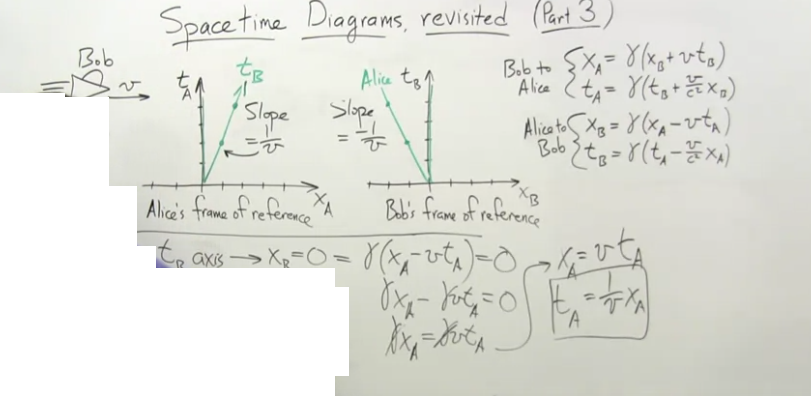Spacetime Diagrams Lorentz Transform.

Recommended Posts

X_B is = 0.  Why do the gamma get crossed out?Edited by can't_think_of_a_name
Share on other sites

Gamma can never be zero.

Share on other sites

xB = γ(xA  -  vtA) = 0

So either γ = 0 or (xA  -  vtA) = 0 or both.

But γ is never zero so

(xA  -  vtA) = 0

Which is not suprising since

xA = xB =0  only when tA = tB = 0

substituting

(0 - v.0) = 0

So sorry but this line of enquiry goes nowhere.

Share on other sites

These notes are from a class. How can this be wrong?

Share on other sites

10 minutes ago, can't_think_of_a_name said:

These notes are from a class. How can this be wrong?

I didn't say was wrong, I said it lead nowhere.

Any straight line through the origin is of the form y = mx where m is the slope.

So you could have written the last line straight down since 1/v is defined as the slope.

But you have also added the condition that xB = 0.

This can only occur at a single point as I noted above.

All your last two lines show is that distance = speed x time or time = distance / speed.

This is pretty common knowledge.

Share on other sites

You have two frames whose origins coincide at time 0. It looks like frame B is moving relative to A at velocity v. The equation x_A = v t_A describes a particle that is fixed to frame B's origin. Alice describes the moving particle to Bob... but it doesn't matter to Bob what gamma is because the particle is at Bob's origin. Eg. whether the length contraction factor is 2 or 3, if the proper length is zero, the relativistic length is still going to be zero.

It could be that the equations are used here to find the equation describing B's origin relative to A. However that seems weird to me, because that form of the Lorentz transformation is based on the specification of how the origins relate, so it's like solving for something already known???

Create an account

Register a new account